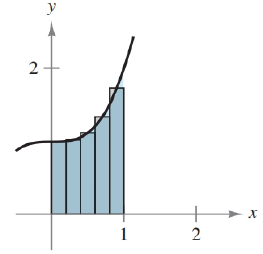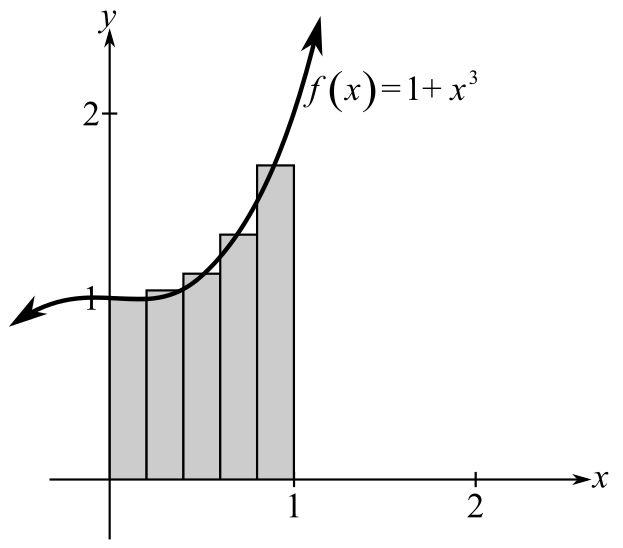Chapter 5.6, Problem 5E### Calculus: An Applied Approach (Min...

10th Edition
Ron Larson
ISBN: 9781305860919

#### Solutions

Chapter
Section### Calculus: An Applied Approach (Min...

10th Edition
Ron Larson
ISBN: 9781305860919
Textbook Problem
1 views

# Approximating the Area of a Plane Region In Exercises 1-6, use the rectangles to approximate the area of the region. Compare your result with the exact area obtained using a definite integral. See Example 1. f ( x ) = x 3 + 1 , [ 0 , 1 ]To determine

To calculate: The area bounded by the function f(x)=1+x3 between interval [0,1] by mid-point rule. Also compare this approximate area with exact area using definite integral.Explanation

Given Information:

The provided function is f(x)=1+x3. Area of this function is calculated between the interval [0,1] as shown below:

Formula used:

The approximate area of any definite integral baf(x)dx by the use of midpoint rule is calculated by the use of following steps.

Step1: First divide the provided interval of the function into n sub intervals by the use of formula;

Δx=ban where [a,b] are the intervals and n is the subinterval value and Δx is the width.

Step2: Use above subinterval find the midpoint of each sub interval.

Step3: Final step is to obtain approximate area by calculating function f at each mid-point from the use of formula;

abf(x)dxban[f(x1)+f(x2)+f(x3)+....+f(xn)].

Calculation:

Consider the provided function f(x)=1+x3. The graph of provided function is shown below:

In provided graph, interval [0,1] is divided into 5 rectangles.

Now, put n=5, a=0 and b=1 to find the width of each rectangle as;

Δx=105=0.2

Width Δx of each rectangle will be 0.2.

So, the interval [0,1] will be divided into 5 subintervals as;

[0,0.2],[0.2,0.4],[0.4,0.6],[0.6,0.8],[0.8,1.0].

Now, the value of midpoint of each rectangle is calculated as;

[x1,x2]=x1+x22 where x1,x2 are the upper value and lower value of the sub-interval

So, for first rectangle [0,0.2] mid-point is;

0+(0.2)2=0.1

Similarly, for second rectangle [0.2,0.4] mid-point is;

0.2+0.42=0.3

For third rectangle [0.4,0.6] mid-point is;

0.4+0.62=0.5

For fourth rectangle [0.6,0.8] mid-point is;

0.6+0.82=0.7

For last rectangle [0.8,1.0] mid-point is;

0.8+1.02=0.9

So, the mid points of all four rectangles are 0

### Still sussing out bartleby?

Check out a sample textbook solution.

See a sample solution

#### The Solution to Your Study Problems

Bartleby provides explanations to thousands of textbook problems written by our experts, many with advanced degrees!

Get Started

#### What is the lowest score in the following distribution?

Essentials of Statistics for The Behavioral Sciences (MindTap Course List)

#### In problems 63-73, factor each expression completely. 73.

Mathematical Applications for the Management, Life, and Social Sciences

#### π does not exist

Study Guide for Stewart's Single Variable Calculus: Early Transcendentals, 8th

#### True or False: converges mean exists.

Study Guide for Stewart's Multivariable Calculus, 8th

#### Define applied research and basic research and identify examples of each.

Research Methods for the Behavioral Sciences (MindTap Course List)